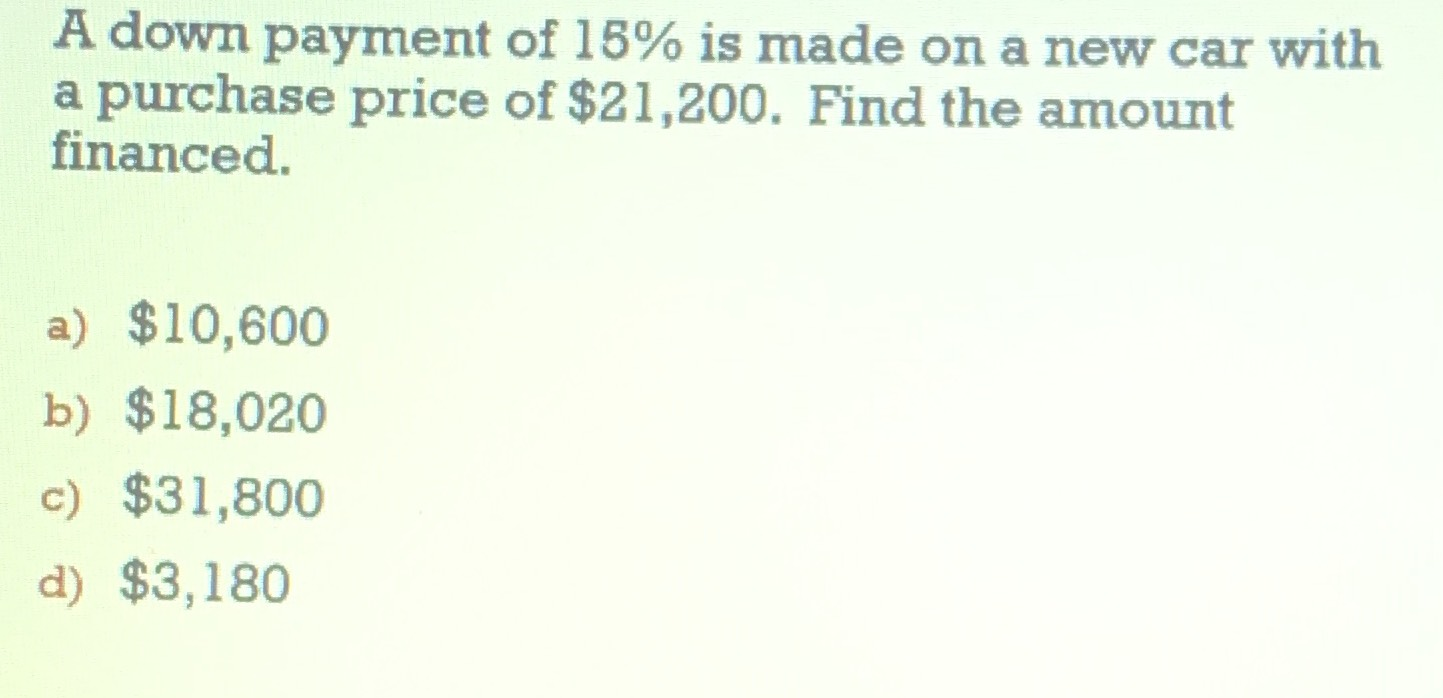### ¿Todavía tienes preguntas de matemáticas?

Pregunte a nuestros tutores expertos
Algebra
PreguntaA down payment of $$15 \%$$ is made on a new car with a purchase price of $$\ 21,200$$ . Find the amount financed.

a) $$\ 10,600$$

b) $$\ 18,020$$

c) $$\ 31,800$$

d) $$\ 3,180$$